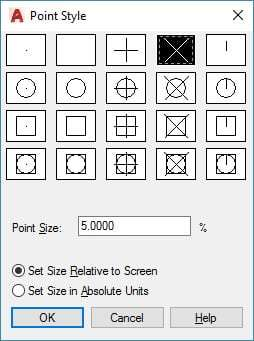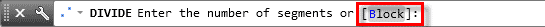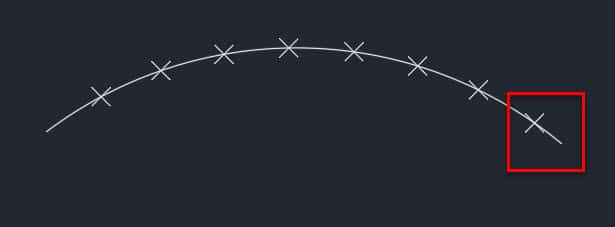Using DIVIDE and MEASURE commands of AutoCAD you can divide 2D drawing objects like Line, Polyline, Arc, Spline and Ellipse in equal parts without knowing their length.

These commands will not split the geometry instead at every division a point will be added. The commands can be used on open geometries like an arc and also on closed geometries like a circle.

## DIVIDE command:

In order to divide the geometry into a fixed number of divisions use DIVIDE command. For our case, I will use a spline.

Select Divide tool from expanded Draw panel of Home tab, you can also use its command equivalent DIVIDE. Click on the spline and enter the number of segments in which you want to divide the spline, I will select 6 segments for our case. Press enter to exit the command.You will see no visible changes on the spline, to see the changes type DDPTYPE and press enter. This will open Point Style window, select a visible point style from this window and click on OK.You will now see points on the spline that divide the entire geometry into six equal segments.

## Blocks for Division:

You can also use blocks instead of points at the division points of the geometry. In order to use the blocks start DIVIDE command select the object and click on Block from options of the command line as shown in the image below.

###Type name of Block and press enter again. If you want to align the object with respect to the geometry select yes from the next prompt else select no. Specify the number of segments in which you want to divide the geometry and press enter.

You will notice that an instance of selected block will be added at the point of division of the geometry.

## MEASURE command:

MEASURE command can be used in the situations where you want to divide the object into segments of known length.

For example, if we have an arc and we want the arc to divide in such a way that each segment is equal to 3.6 units then this command can be used.

However, there is a limitation with this command when you use this command on any geometry the length of the last segment may or may not be equal to the length assigned by you.

The last segment length depends entirely on the remaining length of the curve after performing the divisions as shown in the image below.For example, if you use measure command to divide a line of length 9 units in a segment length of 2 units then the line will be divided into four equal parts of 2 unit length but the last segment will be 1 unit in length.

To use this command select MEASURE tool from expanded draw panel of Home tab, you can also use its command equivalent MEASURE.

Select the geometry and type the length of segment and press enter. You will notice that a node will be added at each division points similar to DIVIDE command. In this case, as well you can use blocks instead of points.

Do you have questions related to this tip? Let me know in the comments below.